# SSAT Upper Level Math : Sequences and Series

## Example Questions

1 2 3 4 5 7 Next →

### Example Question #21 : Nth Term Of An Arithmetic Sequence

Find the next term in the following arithmetic sequence: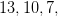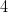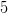Explanation:

The sequence is subtractingfor each term. The next term can be found by subtractingfrom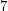: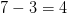### Example Question #21 : Nth Term Of An Arithmetic Sequence

Find the next number in this arithmetic sequence: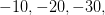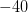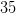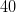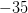Explanation:

The common difference for this arithmetic sequence is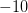. Take away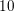from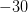to get the next term.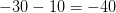### Example Question #551 : Number Concepts And Operations

Find the next term in this arithmetic sequence: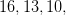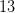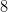Explanation:

The common difference for this sequence is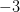. Subtractfrom the last given term to find the next term.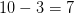1 2 3 4 5 7 Next →

### All SSAT Upper Level Math Resources